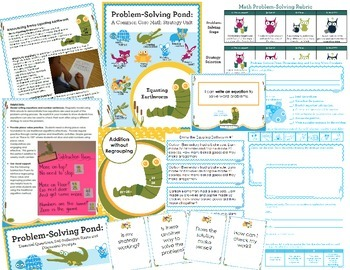# Math Intervention Problem-Solving Unit {Emmy Equating Earthworm}Subject
Resource Type
File Type

Zip

(29 MB|97 pages)
Product Rating
4.0
(7 Ratings)
Standards
• Product Description
• StandardsNEW
Math Intervention: Our CCSS aligned math intervention unit provides 14-20 days worth of differentiated problem-solving activities to give students the strategies they need to solve word problems. Perfect for general education, special education, RTI and math intervention! Can be used with students in grades K-3.

Emmy the Equating Earthworm is a strategy animal in the Problem-Solving Pond series. Emmy uses a special rhyming poem to teach students how to write an equation to solve a word problem. Students can use a variety of strategies to solve equations, including traditional algorithms. Writing an equation is a quick, easy way to double-check solutions for proficient mathematicians.

Emmy's unit includes:

--Introduction to Problem-Solving Pond Unit
--Introduction to Emmy Equating Earthworm
--Suggestions for Use
--Detailed math intervention lesson plans with essential questions
--"I Can" statements
--Problem-solving journal with differentiation and reflection opportunities (perfect for center work, homework or as an assessment)
--Addition and subtraction with and without regrouping
--Essential questions, self-reflection stems and discussion prompts
--3 Problem-solving rubrics
--"Hazel Meets the Math Strategy Animals" book

BEST VALUE - Math Problem Solving Essentials Bundle {K-3}
Contains an entire year's worth of math intervention problem solving lessons and activities with the following strategy animals:

--Modeling Mouse
--Drawing Dragonfly
--Counting Crocodile
--Hopping Hare
--Tabling Turtle
--Equating Earthworm
--Upton Understanding Fish
Use addition and subtraction within 100 to solve one- and two-step word problems involving situations of adding to, taking from, putting together, taking apart, and comparing, with unknowns in all positions, e.g., by using drawings and equations with a symbol for the unknown number to represent the problem.
Understand subtraction as an unknown-addend problem. For example, subtract 10 – 8 by finding the number that makes 10 when added to 8.
Solve word problems that call for addition of three whole numbers whose sum is less than or equal to 20, e.g., by using objects, drawings, and equations with a symbol for the unknown number to represent the problem.
Use addition and subtraction within 20 to solve word problems involving situations of adding to, taking from, putting together, taking apart, and comparing, with unknowns in all positions, e.g., by using objects, drawings, and equations with a symbol for the unknown number to represent the problem.
For any number from 1 to 9, find the number that makes 10 when added to the given number, e.g., by using objects or drawings, and record the answer with a drawing or equation.
Total Pages
97 pages
N/A
Teaching Duration
N/A
Report this Resource to TpT
Reported resources will be reviewed by our team. Report this resource to let us know if this resource violates TpT’s content guidelines.